## Force and Pressure

I. Multiple Choice Questions (MCQs).
Q.1. How do you identify whether a force is acting on a moving object?
a) The object changes direction
b) The object speeds up
c) The object slows down
d) All of these

Q.2. How does frictional force affect the speed of an object?
a) It decreases the speed
b) It increases the speed
c) It has no effect on the speed
d) It sometimes increases and sometimes decreases the speed

Answer. (a) It decreases the speed

Q.3. Mechanical force is an example of
a) contact force
b) non-contact force
c) either (a) or (b)
d) none of these

Q.4. If the area over which the force acts decreases, the pressure
a) Decreases
b) increases
c) both of (a) and (b)
d) none of these

Q.5. A vacuum cleaner is an electrical appliance that cleans surface by
a) absorption
b) blowing
c) suction
d) none of these

II. Fill in the blanks by selecting appropriate words from the given list :

List : atmosphere, motion, force, move, more

Q.1. An interaction of one object with another object results in a……………………….. between the two objects.
Q.2. A force does not always make an object…………………………..
Q.3. A force can change the state of ………………………..
Q.4. The pressure at the bottom of lake is……………………….. than the pressure at the surface.
Q.5. The……………………………. extends upto several kilometres above the surface of the earth.

1- motion,
2- move,
3- motion,
4- more,
5- atmosphere

III. Match the items of Column A with those of Column B.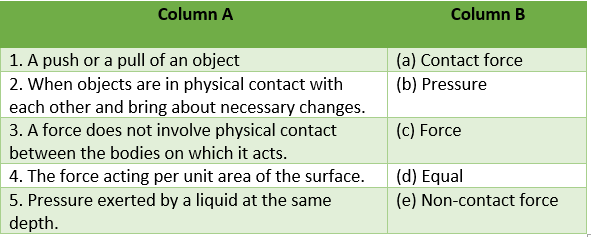Answer. 1-c, 2-a, 3-e, 4-b, 5-d

IV. Write ‘T’ for the true and ‘F’ for the false statements.

Q.1. The direction in which an object is pushed or pulled is called the direction of the force.
Q.2. Force applied in opposite direction decreases speed and force applied in same direction increases speed.
Q.3. The force acting against the relative motion of surfaces in contact is called non-contact force.
Q.4. All objects in the universe exert a force on all other objects.
Q.5. The atmospheric pressure on the earth’s surface at sea level is about 1000 kPa.

Answer: 1-T, 2-T, 3-F, 4-T, 5-T

Q.1. Define force.
Answer: A force is a ‘push’ or ‘pull’ that can change the speed, direction or shape of the body.
OR
A force is defined as an external agency that displaces or tends to displace a body from its position of rest.

Q.2. When you and your friend push an object with same magnitude of force in opposite directions, the object does not move. Why?

Answer: When you and your friend pushed the box in the opposite direction with same force, box does not move. Because in this situation, the net force acting on it is the difference between the two forces. Here the magnitudes of forces are same. i.e., . So, difference – = 0. Hence, box does not move.

Q.3. When a football is kicked, force is applied. Where does the force come from?

Answer. When a football is kicked, force is applied. This force is caused by the action of muscles of our body.

Q.4. When you jump up you come down again. Why?
Ans. When you jump up you come down again due to the gravity.
Q.5. Define pressure.
Ans. The pressure is defined as the force acting per unit area of the surface. Thus,
Pressure = Force/Area

Q.1. Give an example to show how a force changes the state of motion.
Answer: A force can make a stationary body move- when you kick a stationary football it moves. Before being hit, the ball was at rest and so its speed was zero. Goalkeeper also applies a force on the moving ball to stop or deflect the ball, saving a goal. If the goalkeeper succeeds in stopping the ball, its speed decreases to zero.

Q.2. The one wearing the large, flat snow shoes does not sink into the snow. Why?
Answer: Snow shoes stop from sinking into snow because the snow shoes are very large. They have large surface area. According to the Pascal’s formula pressure and surface area are inversely related to each other. So when the surface area is large than the pressure exerted by the shoes will be less on ground. Thus due to this person will not sink into snow.

Q.3. A boy weighing 400 N rests on an area of 100 $cm^{2}$. Calculate the pressure exerted by the boy on the surface which supports it.
Answer: Weight of boy = 400 N
Area = 100 $cm^{2}$ = 0.01 $m^{2}$

Pressure = Force/Area

Pressure 400 N / 0.01 $m^{2} = 40000 Nm^{–2} = 4 × 10^{4} Nm^{–2}$

Q.4. How can you say forces are due to an interaction? Give one example.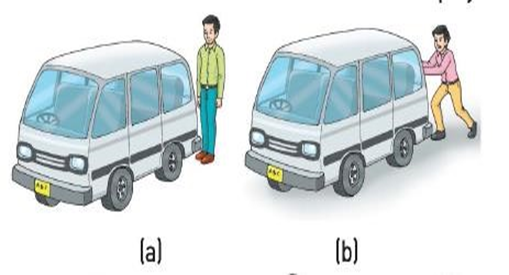Look at the pictures. In fig (a), you see that a man is standing behind a van. In this situation there is no movement in van. In fig (b), you see that man starts pushing the van. In this situation, van moves in forward direction, i.e., in the direction of applied force. In this situation, van moves because man interacts with van. Thus we can say that an interaction of one object with another object results in a force between the two objects.

Q.5. Describe effects of force with a suitable example.
• A force can make a stationary body move- when you kick a stationary football, it moves.
• A force can stop a moving body- A fielder catches a moving cricket ball to stop its motion.
• A force can increase the speed of a moving object- When a force is applied on a moving object –in the direction of motion, its speed increases.
• A force can decrease the speed of a moving object-When a force is applied on a moving object in the direction opposite to the direction of motion, its speed decreases.

Q.6. What do you mean by contact force?
Answer: Contact force is a force which acts only when the objects are in physical contact with each other. Some example of contact forces are muscular force, friction, mechanical force.

Q.1. What do you understand by non-contact force? Describe its kinds with proper examples.
Answer: Non-contact forces do not involve physical contact between the bodies on which they act. They act through the space between them. Such forces are called non-contact forces. They are of four types.

i. Magnetic force : The force which a magnet exerts on magnetic objects is called magnetic force. To know more about this force let us perform this activity.
ii. Electrostatic force : The force exerted by a charged body on another charged or uncharged body is called electrostatic force.

iii. Gravitational force : Newton discovered that all objects in the universe exert a force on all other objects. This is called gravitational force or just gravity. Gravity is not a property of the earth alone. Gravity is an attractive force.

iv. Nuclear force : The force that exists among the tiny particles in the centre(nucleus) of an atom is called nuclear force. It is because of this force that the particles stay together.

Q.2. (a) How does pressure depend on area of contact? Describe it with an activity.
(b) Discuss some examples of pressure in everyday life.

Answer. a) Observe How does pressure depend on area of contact.

Requirements: Two cylinders P and Q of the same weight W. But P is longer and thinner and Q is shorter and thicker, soft clay

Procedure : Spread soft clay on the ground. Now take both cylinders and place both of them on the soft clay as shown in figure. What do you observe? You observe that P is found to penetrate more than Q.

The reason is that the thinner cylinder exerts more pressure than the thicker one. However, the total force exerted by both the cylinders is the same and is equal to W their weight.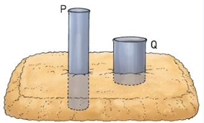The reason is that the thinner cylinder exerts more pressure than the thicker one. However, the total force exerted by both the cylinders is the same and is equal to W their weight.
Pressure exerted by P = W/A , where A is the area of P on which force is exerted and Pressure exerted by Q = W/B where B is the area of Q on which force is exerted and

Since A < B, therefore, pressure exerted by P is greater than that exerted by Q.
Thus we can say that, Pressure = Force/Area ——————(i)
In this expression, force is in numerator and area is in denominator. So, we can draw two conclusion form above equation(i).

• If the force Increases, the pressure increases and vice-versa.
• If the area over which the force acts decreases, the pressure Increases and vice-versa.

b) Examples of pressure in daily life.
• School bags and shopping bags have broad straps or belts so that the area of contact increases and pressure on the hand or shoulder is reduced.
• The desert animals like camels can walk easily on sandy Land compared to other animals because they have broader feet which exert less pressure on the ground.
• The cutting edge of knives, blades, axes, etc., are made sharper. Due to this, the area of contact decreases and hence pressure exerted by these instruments can easily penetrate and cutting becomes easier.
• Rails on a railway track are fixed to large wide wooden or steel sleepers. The heavy thrust of the train on the rail is spread over the large surface area of sleepers. It reduces the pressure on the ground and prevents the rails from sinking into the ground.

Q.3. (a) How can you say that liquid or gas exerts pressure? Describe with an activity.
(b) What do you mean by atmospheric pressure? Discuss some applications of atmospheric pressure in daily life.

Answer. a)With the help of following activities we can say that the liquid exerts pressure.
(i)Liquid exert pressure on its height or depth

A transparent glass tube about 15 cm long and diameter 5–7.5 cm, balloon, water, and iron stand. Take a transparent tube and rubber balloon. Stretch the rubber balloon over one end of the pipe. Clamp the pipe in vertical position in iron stand.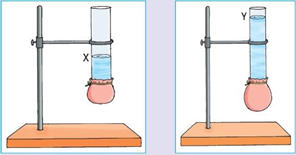Now pour some water in the pipe up to mark x. You observe a small bulge appear in the balloon.
Now pour water upto mark y. You observe that the size of bulge increases.
This is because pressure exerted by water or Liquid at the bottom of the vessel increases with height of its column.
(ii) Liquid exerts pressure on the walls of the container.
Requirements : Discarded soft drink bottle, cylindrical glass tube, rubber balloon, water, molten wax.
Procedure:Take a discarded soft drink bottle. Fix a cylindrical glass tube, a few cm long near its bottom by cutting or slightly heating. Seal it with molten wax to prevent any leakage. Stretch the balloon over the mouth of the cylindrical glass tube.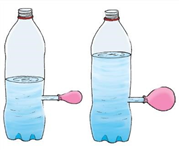Now pour water in the bottle upto half.
You observe that rubber balloon bulge out. Pour some more water in bottle upto ¾ th of its height.
You observe that bulging of the balloon increases.
This is because liquid exerts pressure on the walls of the container (bottle). As the height of the water (liquid) increases, pressure also increases on the walls of the container.

(iii) Liquid exerts equal pressure at the same depth.

Requirements: Discarded soft drink bottle, drill machine, water.

Procedure: Take a discarded soft drink bottle and drill a few equal-sized holes all around it at the same level. Now fill the bottle with water.
You observe that water rushing out of all these falls at the same distance from the wall of the bottle.
This is because liquid exerts equal pressure at the same depth in all directions.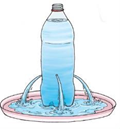From the above activities, it is clear that liquid pressure increases with depth and liquid exert equal pressure at the same depth.

To show that gases exert pressure on the walls of their container we need a bicycle tube, needle, pump.
Procedure: Take a bicycle tube and inflate it with the help of pump. Now puncture it with the help of needle. You observe that gases come out fastly from hole. This is because gases exerts pressure on the inner walls of an inflated tube.

b) The pressure at any point exerted by the weight of the air above it is called atmospheric pressure.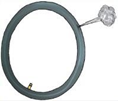Applications of atmospheric pressure in daily life:

(a) Drinking fruit-juice or a cold-drink with straw: The drinking of a Liquid with the help of a hollow straw is based on the pressure exerted by the atmosphere on the surface of the Liquid. When sucked through a hollow straw, the liquid rises into the straw due to difference in the pressure of the air inside and outside the straw.

(b) A vacuum cleaner is an electrical appliance that cleans surfaces by suction. A fan inside the vacuum cleaner lowers the air pressure and creates a low pressure inside the device. Consequently, the air and dirt particles on and near the surface are sucked into the device. This is what helps in cleaning the surface.